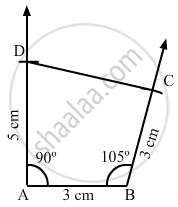Share

# Construct a Quadrilateral Abcd, in Which Ab = Bc = 3 Cm, Ad = 5 Cm, ∠A = 90° and ∠B = 105°. - Mathematics

Course
ConceptConstructing a Quadrilateral When Two Adjacent Sides and Three Angles Are Known

#### Question

Construct a quadrilateral ABCD, in which AB = BC = 3 cm, AD = 5 cm, ∠A = 90° and ∠B = 105°.

#### Solution

Steps of construction:

Step I: Draw AB = 3 cm .

Step II: Construct ∠DAB = 90° at A .

Step III : With A as the centre and radius 5 cm, cut off AD = 5 cm .

Step IV: Construct ∠ABC = 105° at B .

Step V : With B as the centre and radius 3 cm, cut off BC = 3 cm .

Step VI: Join CD .